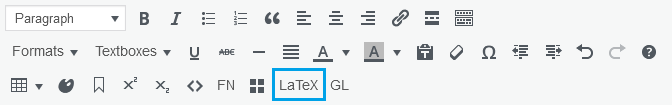# 40 How do I write a LaTeX expression in Pressbooks?

Last update: Jul 12/22

This chapter explains how to write LaTeX expressions in Pressbooks using two different methods.For detailed steps on writing LaTeX in Pressbooks, see Add Mathematical Notation in the Pressbooks User Guide.

# LaTeX shortcodes

Use the shortcodes `$…$` to mark the boundaries between LaTeX and regular text. This is the simplest and best way in Pressbooks to write LaTeX expressions that will be rendered by MathJax. These shortcodes can be used to write inline LaTeX expressions (i.e., in the middle of regular text) or to left-align a LaTeX expression written on its own line.

You can either type these shortcodes yourself or generate them using the LaTeX button on the third row of the visual editor toolbar, seen below:If you are using the British Columbia/Yukon Open Authoring Platform, MathJax will be activated and ready to render whatever you write between these shortcodes into clean, readable mathematical expressions.

For example, the formula $a^2+b^2=c^2$ is better known as the Pythagorean theorem. Here it is again, on its own line and centred using HTML:

$a^2+b^2=c^2$

To see how to write this expression, follow the instructions in How do I view an expression as it appears in LaTeX markup?

# Double dollar sign delimiters

Assuming you’re rendering your LaTeX using MathJax, there are technically two notation methods for writing LaTeX. You can either surround your LaTeX markup with `$…$` or `$$…$$`. However, this second method comes with a catch.

`$$…$$` notation centres a LaTeX expression and puts it on its own line, even if it is used in the middle of a sentence.

For example, the formula $$A=\dfrac{1}{2}bh$$ can be used to calculate the area of a triangle.

In editor view, this looks like `For example, the formula $$A=\dfrac{1}{2}bh$$ can be used to calculate the area of a triangle.`

This is great, except for one major caveat: LaTeX expressions written using `$$…$$` delimiters do not render in PDFs. If you use this notation in the webbook version of your book in Pressbooks, your expression will render and appear as expected. But if you then export the webbook as a PDF, your expression will appear in the PDF as the original LaTeX markup, which is not particularly pretty or human readable.

Therefore, we recommend using `$…$` for all LaTeX expressions if there’s a possibility that you will export your webbook to other formats. If you would like your expression to appear centred and on its own line, simply write it on its own line and centre it using `Shift + Alt + C` or the “Align centre” button on the visual editor toolbar.